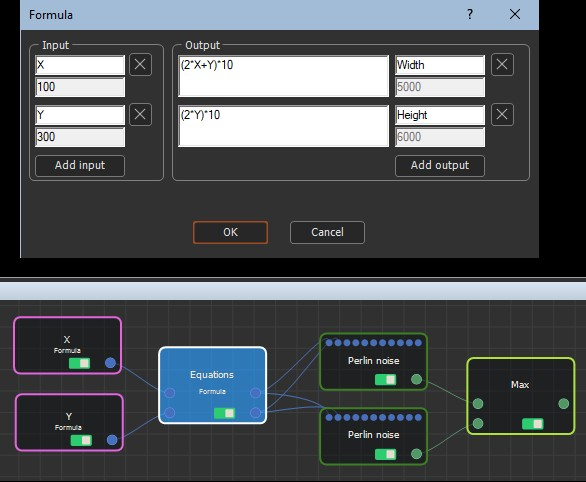The Calculation nodes compute operations on numbers.

• Parameter node: The Parameter node defines the minimum and maximum values of the output value, and the number of decimal places for these values. The output value is then set between the minimum and maximum values using a slider.
• Constant node: The Constant node is a simplified Parameter node. It has one output node that is defined by a value entered in the parameters.
• The following four nodes provide mathematical operations on numbers and have no parameters. They take the first input value of a Calculation node and apply the operation to the second input value of another Calculation node.
- Plus node: Adds the first input value to the second input value.
- Minus node: Subtracts the first input value from the second input value.
- Multiply node: Multiplies first input value with the second input value.
- Divide node: Divides the first input value over the second input value.
• Formula node: The Formula node allows you to create a formula that can be applied to several nodes at the same time.
• File name node: The File name node defines what file to import or export.
• Size information: The Size information node splits the size of the input terrain, mask, color map or normal map and outputs the width in number of vertices, the length in number of vertices, the quad size, the width in meters, and the length in meters.